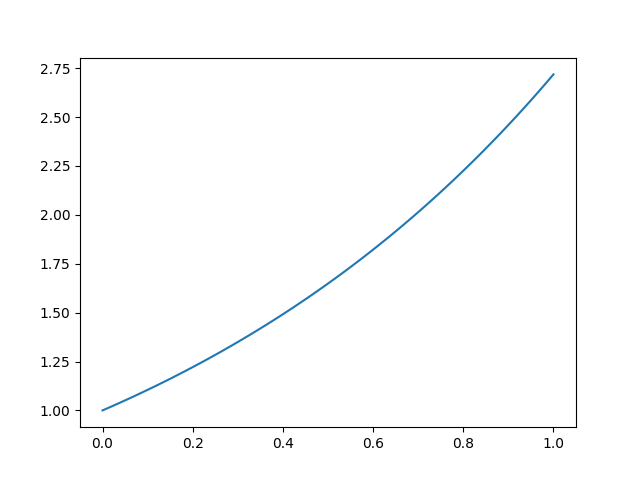# Euler

## Description

Discord bot for typesetting LaTeX and Python programming.

General Utility

# EulerBot

Discord bot for typesetting LaTeX and Python programming.

Invite Euler bot

## LaTeX

• The bot renders messages containg LaTeX code. Any message containing at least a pair of dollar signs $ or \begin will be rendered as a PNG image. • The bot also supports Markdown syntax within$\LaTeX$messages. • The bot can also handle picture or tikzpicture environment. • LaTeX code is rendered by KaTeX. You can specify KaTeX options with the command !katex gistd id where gistid is the id of a public gist containing a JSON object. • Example of a Discord message and the generated image. # Test - Inline math :$x\mapsto x^2$induces a bijection from$\mathbb R_+$onto$\mathbb R_+$. - tikzpicture image \usetikzlibrary{quotes,angles} \begin{tikzpicture}[thick,scale=5, every node/.style={scale=5}] \draw (3,-1) coordinate (a) node[right] {a} -- (0,0) coordinate (b) node[left] {b} -- (2,2) coordinate (c) node[above right] {c} pic["$\alpha\$", draw=orange, <->, angle eccentricity=1.2, angle radius=1cm]
{angle=a--b--c};
\end{tikzpicture}

- Python code

python
def f(x):
return x**2


- Displaystyle math
$$\int_{-\infty}^{+\infty}e^{-t^2}\mathrm{dt}=\sqrt\pi$$• Example of a katex gist
{
"macros": {
"\\cC": "\\mathcal{C}",
"\\cD": "\\mathcal{D}",
"\\cP": "\\mathcal{P}",
"\\la": "\\lambda",
"\\va": "\\varphi",
"\\dF": "\\mathbb{F}",
"\\dK": "\\mathbb{K}",
"\\dN": "\\mathbb{N}",
"\\dQ": "\\mathbb{Q}",
"\\dR": "\\mathbb{R}",
"\\dC": "\\mathbb{C}",
"\\dU": "\\mathbb{U}",
"\\dZ": "\\mathbb{Z}",
"\\lb": "\\left[\\!\\left[",
"\\rb": "\\right]\\!\\right]",
"\\card": "\\operatorname{card}",
"\\vect": "\\operatorname{vect}",
"\\dim": "\\operatorname{dim}",
"\\deg": "\\operatorname{deg}",
"\\ident": "\\operatorname{Id}",
"\\stab": "\\operatorname{Stab}",
"\\Im": "\\operatorname{Im}",
"\\Ker": "\\operatorname{Ker}",
"\\conj": "\\overline",
"\\te": "\\theta",
"\\eps": "\\epsilon",
"\\si": "\\sigma",
"\\al": "\\alpha"
}
}

• The bot's message is updated/deleted whenever the author's message is updated/deleted.

## Python

• Use the !python command to interpret Python code.

• Example of a Python message and generated output

!python
def doubles(l):
return [2*x for x in l]
print(doubles(range(10)))


Code

def doubles(l):
return [2*x for x in l]
print(doubles(range(10)))


Sortie

[0, 2, 4, 6, 8, 10, 12, 14, 16, 18]

• You can use the numpy package.

• You can also the matplotlib package. Each call to show() will generate an image.

• Example of a message using numpy and matplotliband the generated output.

!python
import numpy as np
import matplotlib.pyplot as plt
X=np.linspace(0,1,100)
Y=np.exp(X)
plt.plot(X,Y)
plt.show()


Code

import numpy as np
import matplotlib.pyplot as plt
X=np.linspace(0,1,100)
Y=np.exp(X)
plt.plot(X,Y)
plt.show()


Sortie

pythonAll reviews (0)

Recent reviews (0)

0 invites this week

158 invites total

Prefix: Unknown

Submitted: 04/07/2020

Approved: 06/24/2020

Edited: 04/07/2020

Tip: Sorting your searches by relevance give you more relevant results compared to other sorting options.